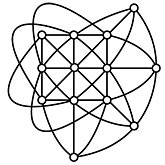# Log24

## Wednesday, August 1, 2012

### Elementary Finite Geometry

Filed under: General,Geometry — Tags: , — m759 @ 7:16 PM

I. General finite geometry (without coordinates):

A finite affine plane of order has n^2 points.

A finite projective plane of order n  has n^2 + n + 1

points because it is formed from an order-n finite affine

plane by adding a line at infinity  that contains n + 1 points.

Examples—II. Galois finite geometry (with coordinates over a Galois field):

A finite projective Galois plane of order n has n^2 + n + 1

points because it is formed from a finite affine Galois 3-space

of order n with n^3 points by discarding the point (0,0,0) and

identifying the points whose coordinates are multiples of the

(n-1) nonzero scalars.

Note: The resulting Galois plane of order n has

(n^3-1)/(n-1)= (n^2 + n + 1) points because

(n^2 + n + 1)(n – 1) =

(n^3 + n^2 + n – n^2 – n – 1) = (n^3 – 1) .

III. Related art:

Another version of a 1994 picture that accompanied a New Yorker
article, "Atheists with Attitude," in the issue dated May 21, 2007:The Four Gods  of Borofsky correspond to the four axes of
symmetry
of a square and to the four points on a line at infinity
in an order-3 projective plane as described in Part I above.

Those who prefer literature to mathematics may, if they like,
view the Borofsky work as depicting

"Blake's Four Zoas, which represent four aspects
of the Almighty God" —Wikipedia